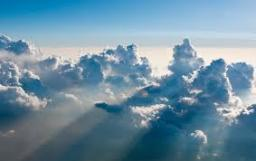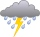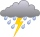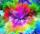# Rainfall

How many liters of water did fell in a 32m long and 8m wide garden, if 8mm of rain fell?

x =  2048 l

### Step-by-step explanation:Did you find an error or inaccuracy? Feel free to write us. Thank you!Tips to related online calculators
Do you want to convert area units?
Do you know the volume and unit volume, and want to convert volume units?

#### You need to know the following knowledge to solve this word math problem:

We encourage you to watch this tutorial video on this math problem:

## Related math problems and questions:

• RainfallOn Thursday, fell 1 cm rainfall. How many liters of water fell to rectangular garden with dimensions of 22 m x 35 m?
• RainGarden shape of a rectangle measuring 15 m and 20 m rained water up to 3 mm. How many liters of water rained in the garden?
• Annual rainfallThe average annual rainfall is 686 mm. How many liters will fall on the 1-hectare field?
• RainfallA rectangular garden of 25m in length and width 20m in width fall 4mm of water. Express by a fraction in basic form what part of the 60-hectolitre tank we would fill with this water.
• Water levelHow high is the water in the swimming pool with dimensions of 37m in length and 15m in width, if an inlet valve is opened for 10 hours flowing 12 liters of water per second?
• The tankThe tank has 1320 liters of water. The tank has the shape of a prism, its base is an rectangle with sides a = 0,6 m and b = 1,5 m. How high does the water level reach in the tank?
• RainfallAnnual rainfall in our country is an average of 797 mm. How many m3 of water rains on average per hectare?
• Rainwater6 mm of water rained on the garden with an area of 25 acres. How many 12 liter cans of water would we water this garden just as much?
• Cuboid aquariumCuboid 25 times 30 cm. How long is third side if cuboid contains 30 liters of water?
• Water tank300hl of water was filled into the tank 12 m long and 6 m wide. How high does it reach?
• GardenGarden with an area of 800 square meters rained 3 mm of water. How many 10-liter cans of water is needed to same irrigation?
• RainfallThe annual average rainfall in India was in Cherrapunji in the year 1981 26 461 mm. How many hectoliters of water fell on 1 m2? Would fit this amount of water into a cube of three meters?
• AquariumCan 30 liters of water fit in a cuboid aquarium with dimensions a = 3dm b = 6dm c = 5dm?
• Water reservoirThe water tank has a cuboid with edges a= 1 m, b=2 m , c = 1 m. Calculate how many centimeters of water level falls, if we fill fifteen 12 liters cans.
• Surface and volume od cuboidContent area of the square base of cuboid is Sp = 36 cm2 and its height 80 mm. Determine its surface area and volume.
• How manyHow many cans of blue paint need to be bought if the interior of the garden pool, which is 5 m long, 3 m wide and 1 m deep, is to be painted? There is 1 kg of paint in each can. One can is enough for 8 m2 of area.
• Children's poolChildren's pool at the swimming pool is 10m long, 5m wide and 50cm deep. Calculate: (a) how many m2 of tiles are needed for lining the perimeter walls of the pool? (b) how many hectoliters of water will fit into the pool?